## Topic 16.1: Simplex Method (HOWTO)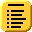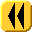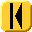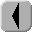Introduction Notes Theory HOWTO Examples Engineering Error Questions Matlab Maple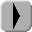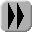# Problem

Given the linear objective function

f(x) = c1x1 + c2x2 + ⋅⋅⋅ + cnxn

subject to a system of system of m linear constraints in n variables, where mn:

a1,1 x1 + a1,2 x2 + ⋅⋅⋅ a1,n xnb1
a2,1 x1 + a2,2 x2 + ⋅⋅⋅ a2,n xnb2

am,1 x1 + am,2 x2 + ⋅⋅⋅ am,n xnbm

and requiring that each variable is positive

x1 ≥ 0
x2 ≥ 0

xn ≥ 0

find a value x which maximizes the function and satisfies the all of the given constraints.

We may write this as maximizing f(x) = cTx subject to Axb and x ≥ 0.

# Assumptions

The rank of the matrix B is M.

# Tools

We will use linear algebra and iteration.

# Initial Conditions

Define the matrix

where Idm is the m × m identity matrix and 0m is an m-dimensional column vector. This is an (n + m + 1) × (m + 1) matrix.

Note that the bottom right entry is a simple zero. This is the value of the objective function f at the point x = 0.

The entries of the bottom row (excluding the last entry) are termed indicators. A negative value in this location will indicate that an increase in the variable associated with that column will result in a positive increase in the objective function.

# Iterative Process

Select a column j such that the indicator is negative. Select the row i (not including the last) which at the least increases the jth variable (divide the last column by the entry in the jth column choose the smallest positive entry, ignoring any division-by-zeros) to be the pivot.

Perform Gaussian elimination on the jth column using row i as the pivot (add the appropriate multiples of row i to all other rows (including the last row).

For instructive purposes, it is most useful to reduce the matrix to row-reduced echelon form.

Continue doing so until all indicators are positive.

# Terminating Conditions

We finish when all indicators are positive. Assuming row-reduced echelon form was used, for each variable x1 through xn for which the column is still in reduced-echelon form read off the value in the last column of the row containing the 1. Otherwise, set the variable to 0.